# Embedded figures test-2

1) From the given answer figures, select the one in which the question figure embedded?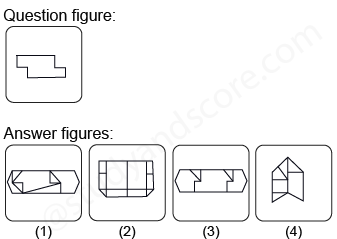2) From the given answer figures, select the one in which the question figure embedded?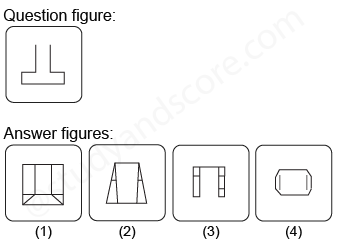3) From the given answer figures, select the one in which the question figure embedded?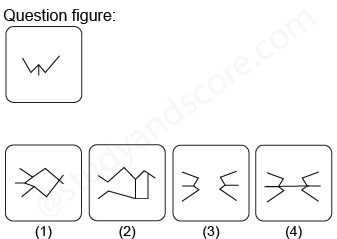4) From the given answer figures, select the one in which the question figure embedded?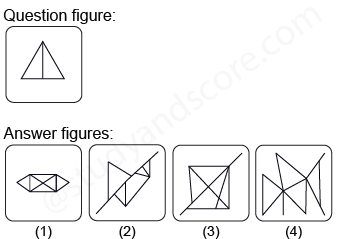5) From the given answer figures, select the one in which the question figure embedded?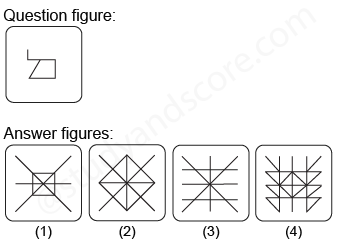--Share with your friends --